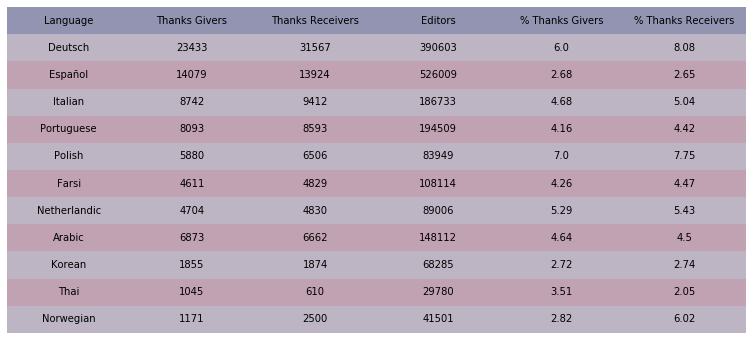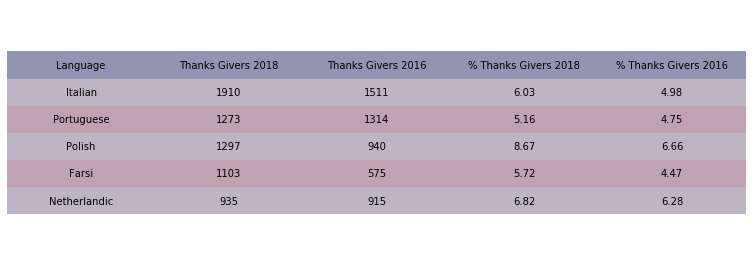#### Introduction¶

The code for making the thanker network data tables is contained in this notebook. These tables provide information on thanks usage rates as well as the size of the thanker/receiver community.

#### (1) Get Thanks Givers¶

• returns the number of people who gave a thank

use PROJECT;

select count(distinct log_user_text) from logging_userindex where (log_action = 'thank' and log_type='thanks' and log_timestamp < timestamp('2018-06-01') and log_timestamp >= timestamp('2013-06-01'))

#### (2) Get Thanks Receivers¶

• returns the number of people who received a thank

use PROJECT;

select count(distinct log_title) from logging_userindex where (log_action = 'thank' and log_type='thanks' and log_timestamp < timestamp('2018-06-01') and log_timestamp >= timestamp('2013-06-01'))

Note: log_user_text and log_title are usernames, not IDs, which is why some studies will have workarounds for potential bugs relating to username changes. Some studies may also use log_user instead of log_user_text. The reason this study uses log_user_text is that there is no ID equivalent of log_title and it's important for the data to be consistent between thanks given and thanks received.

#### (3) Get Editors¶

use PROJECT;

select count(distinct rev_user) from (select rev_user, count(rev_user) as num_edits from revision where (rev_user != 0 and rev_timestamp < timestamp('2018-06-01') and rev_timestamp >= timestamp('2013-06-01')) group by rev_user) as A

There are two analyses in this notebook. The first uses timeframes of five years (June 2013-June 2018), which is essentially the entire time for which the thanks feature has existed. The second uses timeframes of 6-months (either Jan-July 2016 or Jan-July 2018).

If you want to look into the data with the total editor count being only those who have made 5+ edits, go to the Project Personal/Backups directory. If that statement doesn't seem relevant to you, ignore it.

import csv

#define filenames
src = '(1-1)-data/'
filenames = ['thanks-reach-sample.csv', 'thanks-usage-sample.csv']

input_files = [src+filename for filename in filenames]

#define shape of data
data1 = [*4] * 11
data2 = [*5]*5


Note: The SQL queries will return csvs with a single number. To use this pipeline, you will have to manually amalgamate the data.

#get data from csv (which was manually created)
def get_data(data, input_file):
i = 0
with open(input_file, 'r', encoding = 'utf-8') as csvfile:
for row in rder:
data[i] = [row[k] for k in row]
for j in range(1, len(data[i])):
data[i][j] = int(data[i][j])
i += 1

get_data(data1, input_files)

get_data(data2, input_files)


Note: data1 and data2 hold different information

#add percentage columns to data1
for i in range(0, len(data1)):
data1[i] = data1[i] + [data1[i]*100.0/data1[i], data1[i]*100.0/data1[i]]

#convert some columns of data2 to percentages
for i in range(0, len(data2)):
data2[i] = data2[i]*100.0/data2[i]
data2[i] = data2[i]*100.0/data2[i]

import numpy as np
import pandas as pd
import matplotlib.pyplot as plt

#define columns for table
columns1 = ['Language', 'Thanks Givers', 'Thanks Receivers', 'Editors', '% Thanks Givers', '% Thanks Receivers']
columns2 = ['Language', 'Thanks Givers 2018', 'Thanks Givers 2016', '% Thanks Givers 2018', '% Thanks Givers 2016']

#define titles -- used to name table files
title1 = 'thank-users-population'
title2 = 'thanks-usage-rates'

def show_table(data=data1, columns=columns1, title=title1):
fig, ax = plt.subplots()

#hide axes
ax.axis('off')
ax.axis('tight')

#styling -- color cells by row, round all floats
colors = [['#c1a2b2']*len(data)]*len(data)
for i in range(0, len(colors)):
if (i % 2) == 0:
colors[i] = ['#bdb4c4']*len(data)
for i in range(0, len(data)):
for j in range(1, len(data[i])):
data[i][j] = round(data[i][j], 2)

df = pd.DataFrame(data, columns=columns)

table = ax.table(bbox=None, cellText=df.values, cellColours=colors, colColours=['#9294b2']*len(columns), colLabels=df.columns, loc='center', cellLoc='center')

#styling -- get rid of lines in table
d = table.get_celld()
for k in d:
d[k].set_linewidth(0)

fig.tight_layout()
table.scale(2, 2)

plt.savefig('../figures/'+title+'.png', bbox_inches='tight')
plt.show()

show_table(data1, columns1, title1)show_table(data2, columns2, title2)#### Conclusions¶

• The majority of editors have never been touched by the thanks feature, but the number that have is sufficient for us to do good analysis.
• The usage rate of thanks has increased in the past few years.